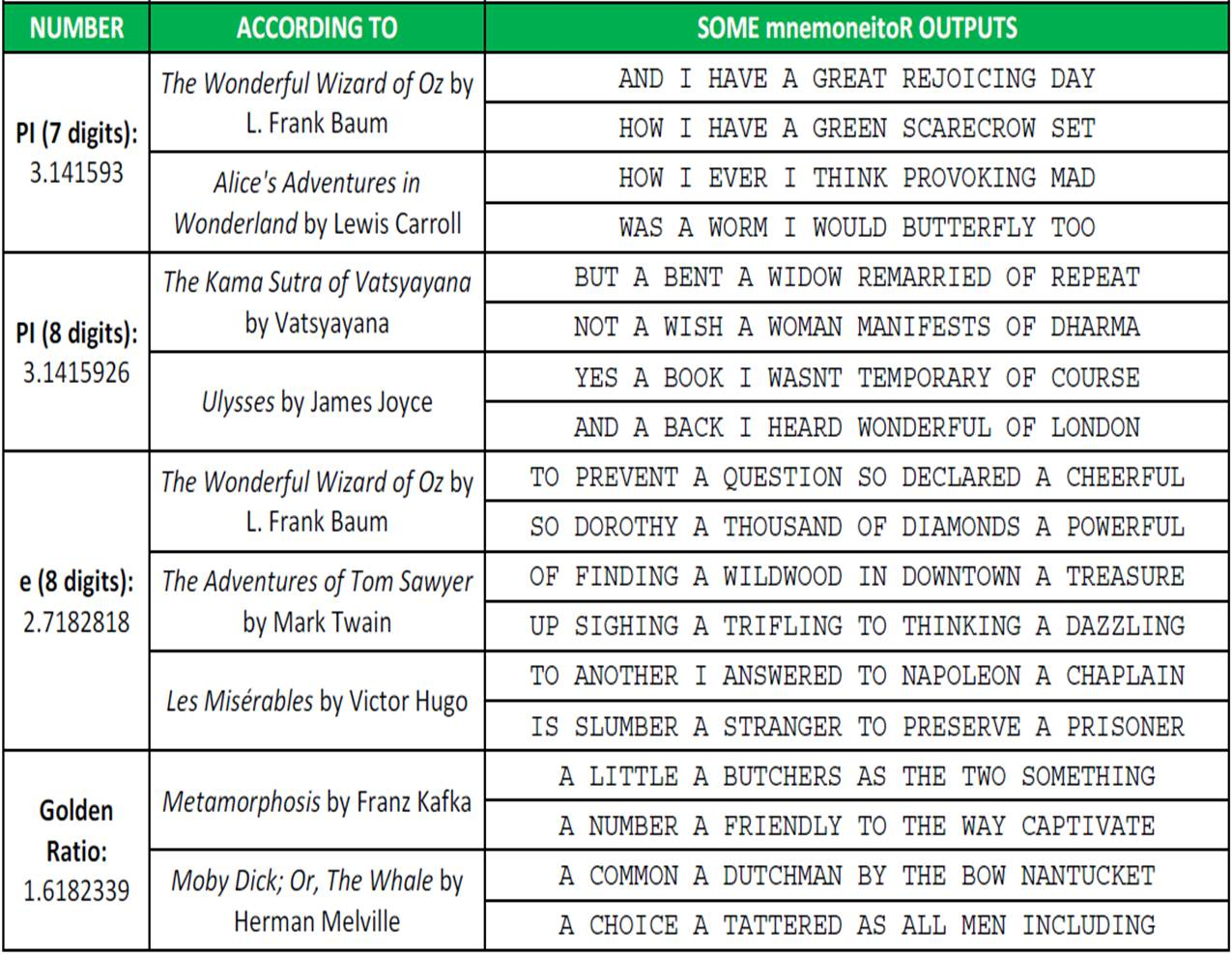# The mnemoneitoR

AND I HAVE A GREAT REJOICING DAY (mnemonic rule generated by mnemoneitoR for first 7 digits of Pi according to The Wonderful Wizard Of Oz)

Is there some number impossible to memorize? Do not worry, here comes mnemoneitoR: the tool that you was always looking for! With mnemoneitoR you can translate any number into an easy-to-remember phrase inspired by your favorite book. It is very easy: choose a book, enter the number and mnemoneitoR will show you as many possibilities as you want. Just choose the one you like most!

There are many webs about mnemonics in the Internet, like this one. One of my favourite menmonic devices for Pi is:

HOW I WANT A DRINK, ALCOHOLIC OF COURSE, AFTER THE HEAVY LECTURES INVOLVING QUANTUM MECHANICS

The number of letters in each word gives the respective number in the sequence (i.e., 3.14159265358979).

For professional purposes, I am learning how to manage texts in R and I discovered a very useful package called `stringr`. This is the only one I need for this experiment. The process is simple: I download a book from Project Gutenberg site, clean and split the text and do simulations on the fly of a Markov Chain generated from the words of the book. Step by step:

• Downloading the book is quite simple. You search the one you want, copy the url in the code (after line “CHOOSE YOUR FAVORITE BOOK HERE”) and no more.
• After loading the text, some easy tasks are needed: remove header and footer lines, split text into words, turn them into uppercase, remove non-text characters … typical things working with texts.
• After reading the number you want to translate, I choose a word sampling along all words with the same number of letters as the first digit with probability equal to the number of appearances. This is how I initialize the phrase. Next word are chose among the set of words which are preceded by the first one and have the same number of letters as the second digit with probability equal to number of appearances, and so on. This is a simulation on the fly of Markov Chain because I do not have to calculate the chain explicitly.
• I always translate Zero with the same word you choose. I like using “OZ” instead Zero.

Most of the phrases do not have any sense but are quite funny. Few of them have some sense and maybe with a small tweak, can change into full of meaning sentences. Here you have some samples of the output of mnemoneitoR:I like how the phrases smell like the original book. I will try to improve mnemoneitoR in the future but I can imagine some uses of this current version: message generator for fortune cookies,  a cool way to translate your telephone number into a sentence …

Here you have the code. If you discover nice outputs in your experiments, please let me know:

```library(stringr)
# CHOOSE YOUR FAVORITE BOOK HERE (Currently "The Wonderful Wizard of Oz")
TEXTFILE = "data/pg55.txt"
# Remove header and footer, concatenate all of the lines, remove non-text and double spaces chars and to upper
textfile = textfile[(grep('START OF THIS PROJECT', textfile, value=FALSE)+1:grep('END OF THIS PROJECT', textfile, value=FALSE)-1)]
textfile <- paste(textfile, collapse = " ")
textfile <- gsub("[^a-zA-Z ]","", textfile)
textfile <- toupper(textfile)
textfile <- gsub("^ *|(?<= ) | *\$", "", textfile, perl=T)
# Split file into words
textfile.words <- strsplit(textfile," ")
textfile.words.freq <- as.data.frame(table(textfile.words));
names(textfile.words.freq) <- c("word", "freq")
textfile.words.freq\$length <- apply(data.frame(textfile.words.freq[,c("word")]), 1, function(x) nchar(x))
# ENTER YOUR NUMBER HERE!!!!!!
number <- 3.1415926
number <- gsub("[^0-9]","", as.character(number))
# Define the word representing Zero
zero.word = "OZ"
fg <- as.integer(substr(number, 1, 1))
df <- textfile.words.freq[textfile.words.freq\$length==fg,]
wd <- sample(df\$word, size=1, prob=df\$freq)
phrase <- c(as.character(wd))
for (j in 2:nchar(number))
{
fg <- as.integer(substr(number, j, j)) if (fg>0)
{
lc <- as.data.frame(str_locate_all(textfile, as.vector(paste(wd, " ", sep = ""))))
lc\$char <- apply(lc, 1, function(x) substr(textfile, as.integer(x)+1+fg, as.integer(x)+1+fg))
fq <- as.data.frame(table(apply(lc[lc\$char==" ",], 1, function(x) substr(textfile, as.integer(x)+1, as.integer(x)+fg))))
if (nrow(fq)==0) fq <- data.frame(word= character(0), freq= integer(0))
names(fq) <- c("word", "freq")
fq\$length <- apply(fq, 1, function(x) nchar(gsub(" ","", x)))
fq <- fq[fq\$length==fg,]
wd <- if(nrow(fq)>0) sample(fq\$word, size=1, prob=fq\$freq)
else
{
df <- textfile.words.freq[textfile.words.freq\$length==fg,]
wd <- sample(df\$word, size=1, prob=df\$freq)
}
}
else wd <- zero.word
phrase <- c(phrase, as.character(wd))
}
print(paste(phrase, collapse = " "))
```